Published on: 2020-07-09

# Experiments with confidence determining networks

This is a followup article to Confidence in machine learning, reading that one first is probably require in order to understand some of the context behind these experiments. That being said, I think the results are potentially interesting on their own.

## I Experimental setup

All the code and data can be found here: https://github.com/George3d6/Confidence-Determination

If you want to but are unable to replicate some of this let me know. Everything random should be statically seeded so unless something went wrong you'd get the exact same numbers I did.

To back up my ruminations on the idea of confidence determination I will do the following experiment:

Take a fully connected network (`M`) and train it on 4 datasets:

The first dataset (a) is a fully deterministic dataset dictated by some n-th degree polynomial function:

`f(X) = c1 \* X^1 + ... cn * X[n]^n`

Just to keep it simple let's say `ck = k or ck = (n+1-k)`

We might also play with variation of this function where we remove the power or the coefficient (e.g. `f(X) = 1*X...n*X[n]` and `f(X) = X^1 + ... X[n]^n`). The choice is not that important, what we care about is getting a model that converges almost perfectly in a somewhat short amount of time.

We might also go with an even simpler "classification" version, where `f(X) = x % 3 if x < 10 else None for x in X`, thus getting an input of only 0, 1 and 2. Whichever of these 3 labels is more common, that's the value of `Y`.

The value to be predicted, `Y = 0 | f(x) < lim1, 1 | lim1 < f(x) < lim2, 2 | lim2 < f(x)`

lim1 and lim2 will be picked such that, for randomly generate values in X between 1 and 9, the split between the potential values of Y in the dataset is 33/33/33.

The second dataset (b) is similar to (a) except for the value of Y, which is:

`Y = 0 | f(x) < lim1, 1 | lim1 < f(x)`

But, given some irregularity in the inputs, say `X == 5 => Y = 2`

The value of `lim1` will be picked such that the initial split is 50/50, given that we are using the 1st-degree variable to introduce this irregularity in 1/9th of samples, I assume the final split will be around 11/44.5/44.5.

The third dataset (c) is similar to (a) except that when Y == 2 there's a 1/3 chance that Y will randomly become 0.

These 3 datasets are symbolic, though not fully representative, of 3 "cases" in which I'd like to think about the idea of confidence:

a) Represents a case where the datasets follow a straight forward equation which, in theory, the model should be able to approximate with ~100% accuracy.

b) Represents a case where the datasets follow a deterministic equation, but this equation has an irregularity which breaks its linearity in several places, which seem "random" when looking at the final value but obvious when looking at the input independently.

c) Represents a case where noise is added to the data, such that 100% accuracy can't be reached. However, assuming a perfect model, we know the confidence we should have in our predictions: `P(correct|1) = 1, P(correct|0) = 75, P(correct|2) = 66`.

I want to test some solutions here and evaluate them based on six criteria:

1. Accuracy, overall accuracy achieved on a testing datasets generated by the same process as the original ones.

2. Given that I only pick predictions with high confidences (say 0.8 quantile), how high is the accuracy for this subset of predictions?

3. Given that I only pick predictions with above-average confidences (say 0.5 quantile), how high is the accuracy for this subset of predictions?

4. Given that I only all pick predictions but those with the lowest confidences (say 0.2 quantile), how high is the accuracy for this subset of predictions?

5. Given a random subset of predictions + confidences, is the mean of the confidence values equal to the accuracy for that subset of predictions?

6. same as (4) but on the whole dataset rather than a random subset.

7. Assuming a situation where a wrong prediction is `-1` and a correct prediction `1`, and the accuracy score is the sum of all these `-1` and `1` values. Would weighting this value by their associated confidence increase the value of this sum?

8. How long does the setup take to converge on a validation dataset? For ease of experimenting, this means "how long does the main predictive model take to converge", once that converges we'll stop regardless of how good the confidence model has gotten. This will also be capped to avoid wasting too much time if a given model fails to converge in a reasonable amount of time compared to the others.

9. The number of epochs it takes each model to converge.

The models evaluated will be:

• Just letting `M` predict, no confidence involved.

• Let `M` predict and have a separate model `C` that predicts the confidence (on a scale from 0 to 1) based on the inputs `X` and `Yh`.

• Let a different model `M'` predict, this is a model similar to `M` but it includes an additional cell into the outputs for predicting a confidence value and some additional cells evenly distributed in all layers in order to have an `M'` training in roughly equal time to that of `M` + `C`.

• Let `M` predict and have a separate model `C` that predicts the confidence just like before, however, the loss from the `Yh` component of the last layer of `C` is backproped through `M`.

I will run each model through all datasets and compare the results. Running model `M` alone serves as a sanity benchmark for the accuracy values and is there in order to set the maximum training time (4x how long it take to "converge" on validation data) to avoid wasting too much time on setups that are very slow to converge (these would probably be impractical).

I will also use multiple datasets, the combinations will be:

1. Degree: 3 ,4 ,5 ,and 6

2. Function: linear, polynomial, and polynomial with coefficients

For each dataset, I will generate a training, validation, and testing set of equal size, consisting of either ~1/3 of all possible combinations or 10,000 observations (whichever is smaller), without any duplicate values in `X`.

Barring issues with training time I will use the 6th-degree polynomial with coefficients as my example, as it should be the most complex dataset of the bunch.

Though, I might use the other examples to point out interesting behavior. This is not set in stone, but I think it's good to lay this out beforehand as to not be tempted to hack my experiment in order to generate nicer looking results, I already have way too many measures to cherry-pick an effect out of anyway.

## II The results

A few days later, I have some results:

Here they are (for the 6th degree polynomial with coefficients dataset):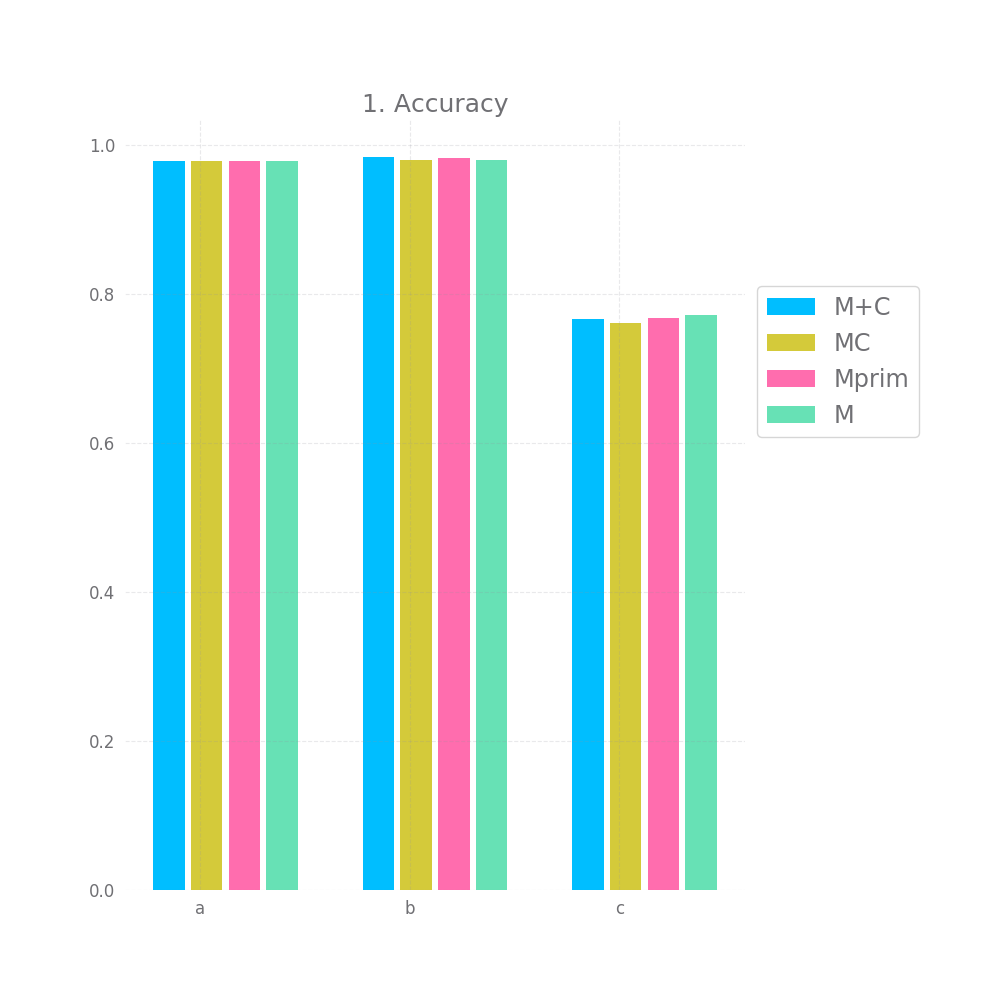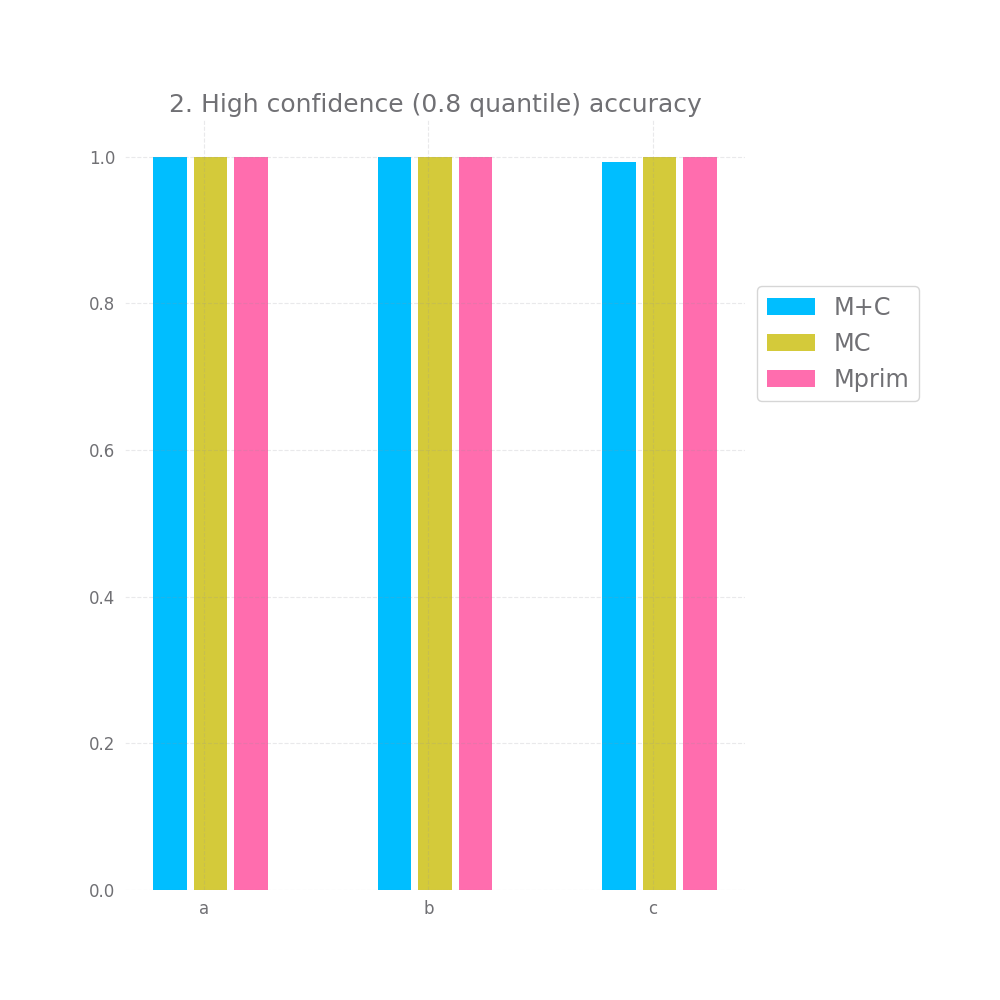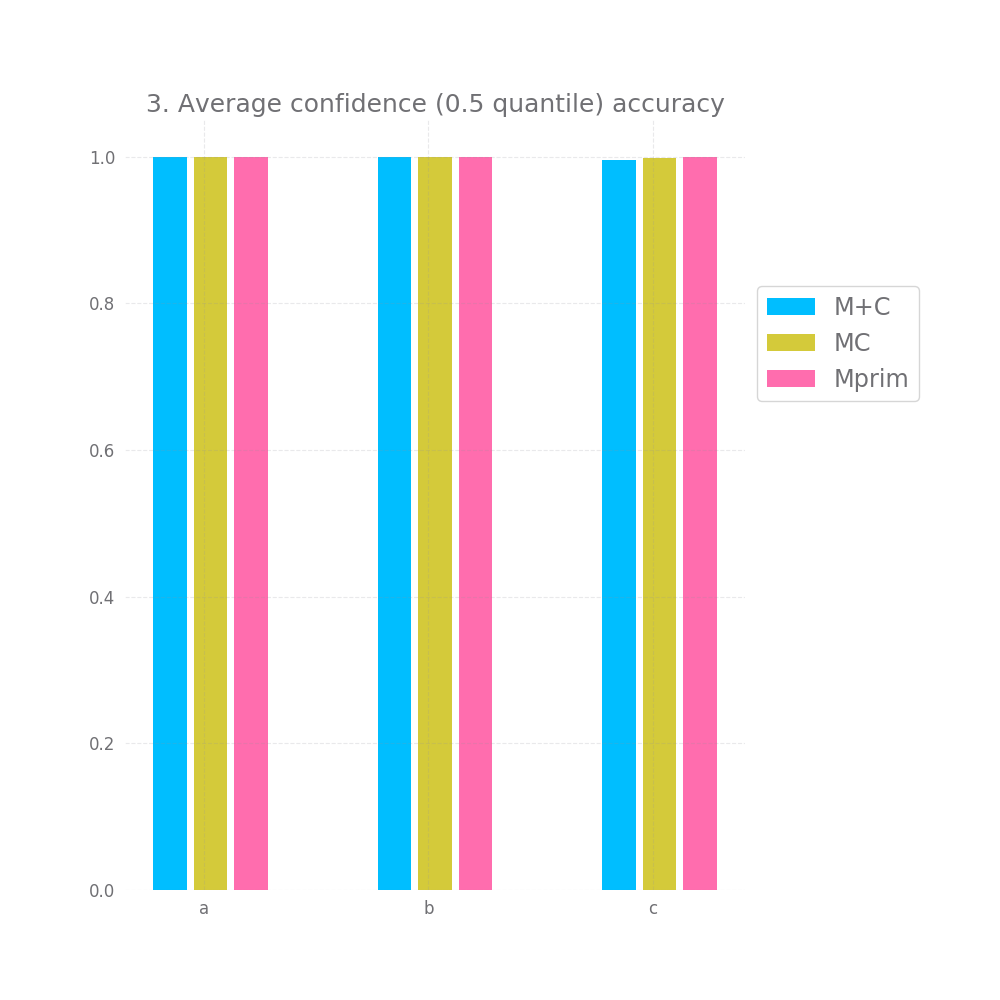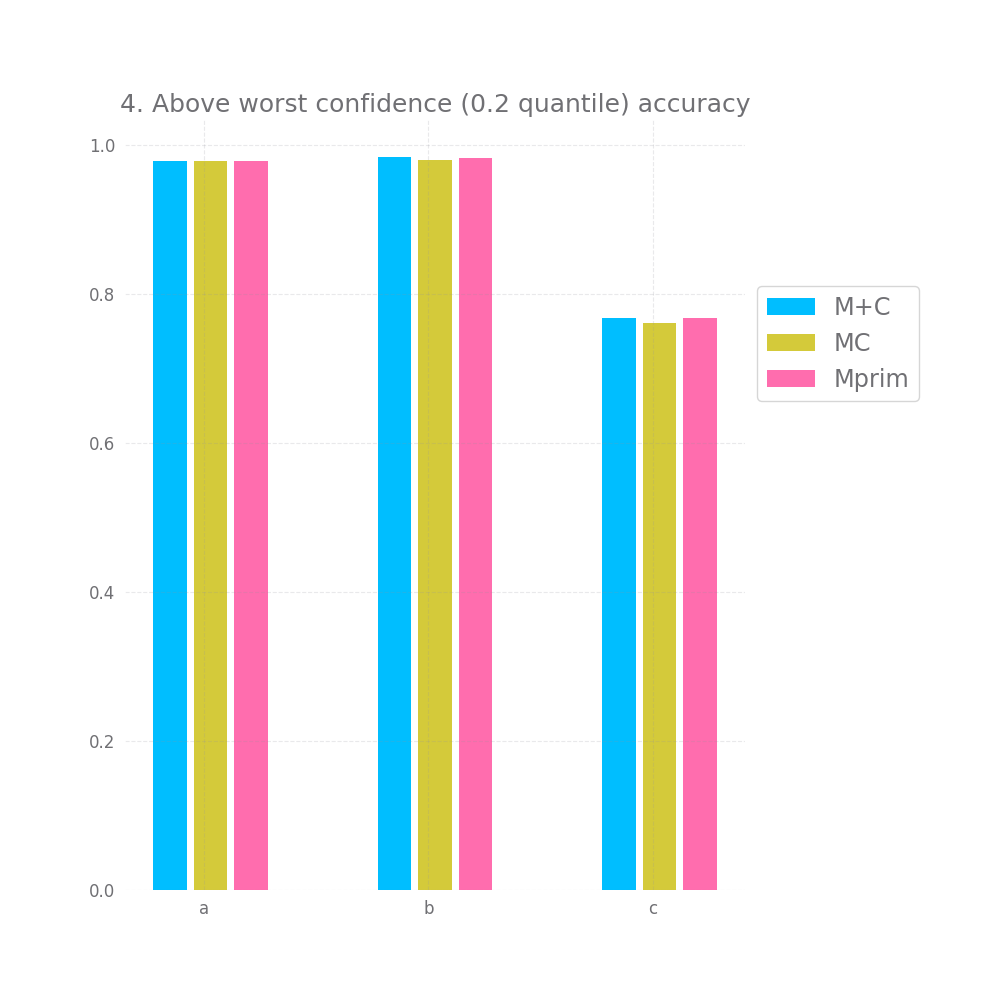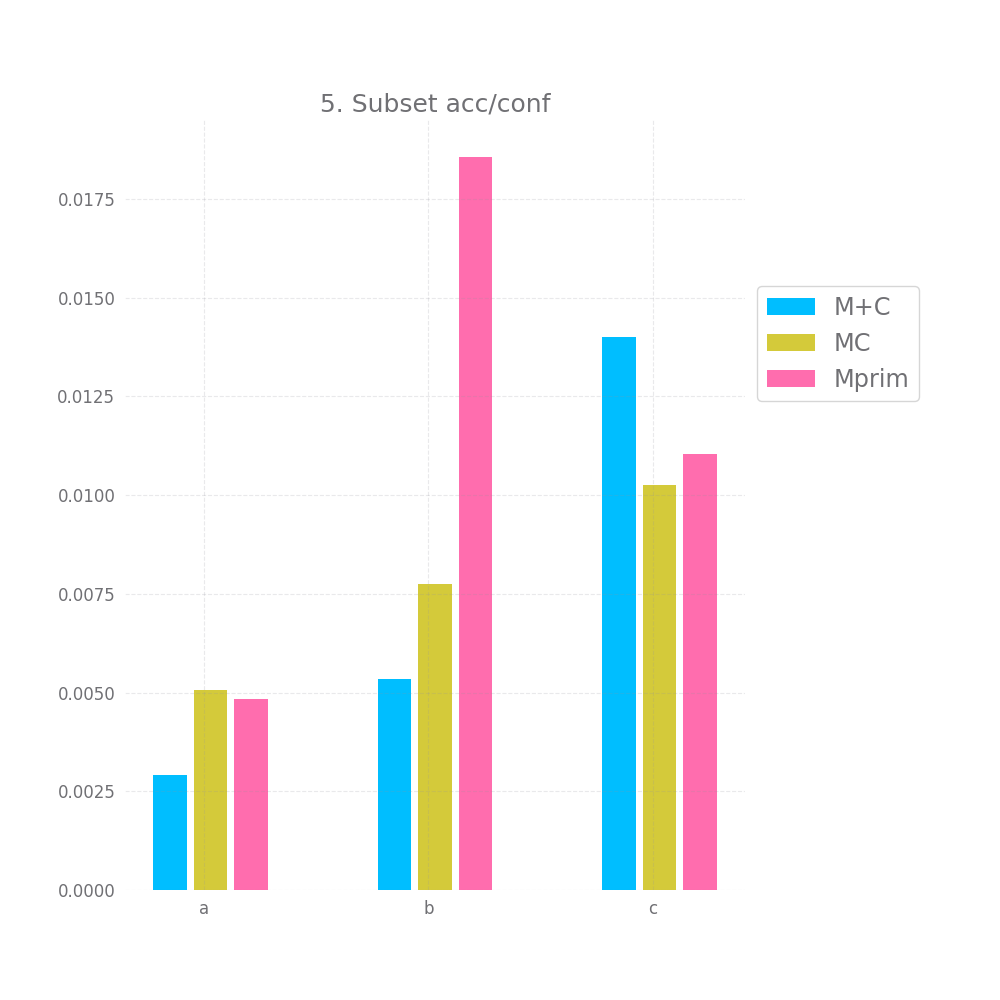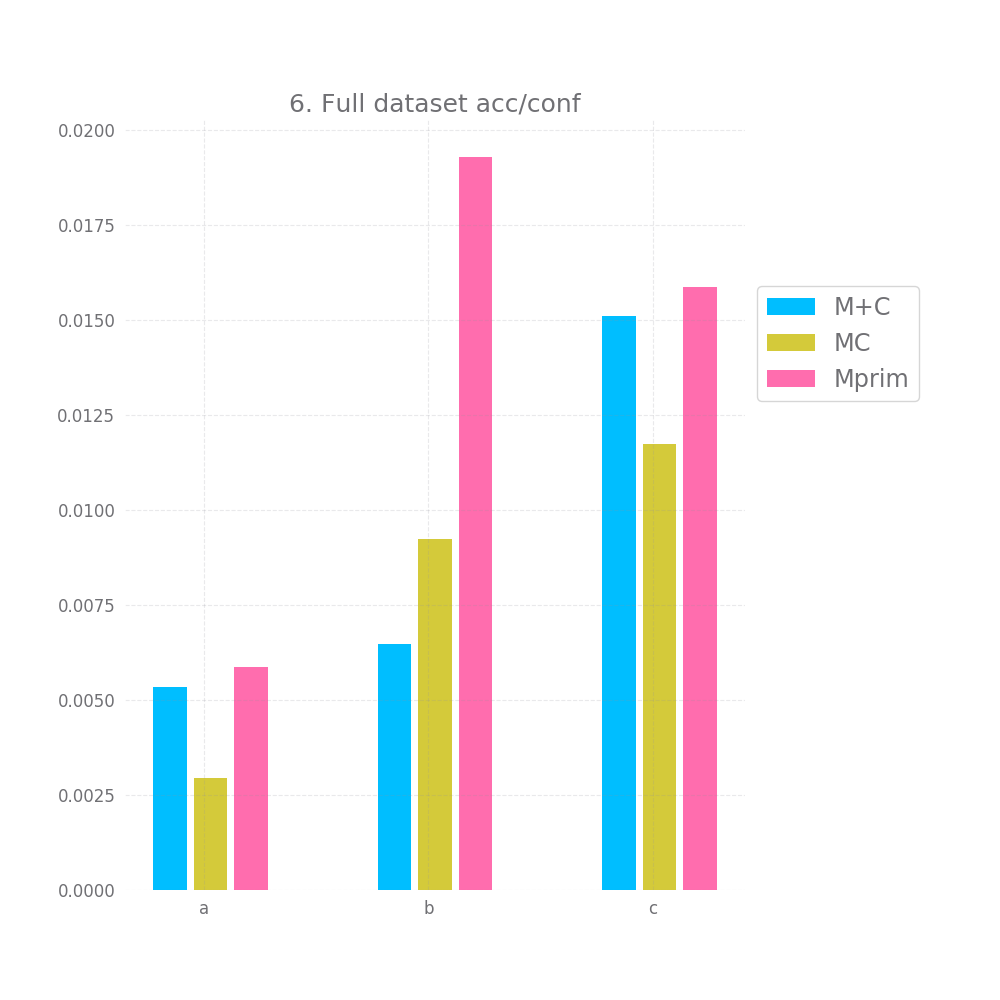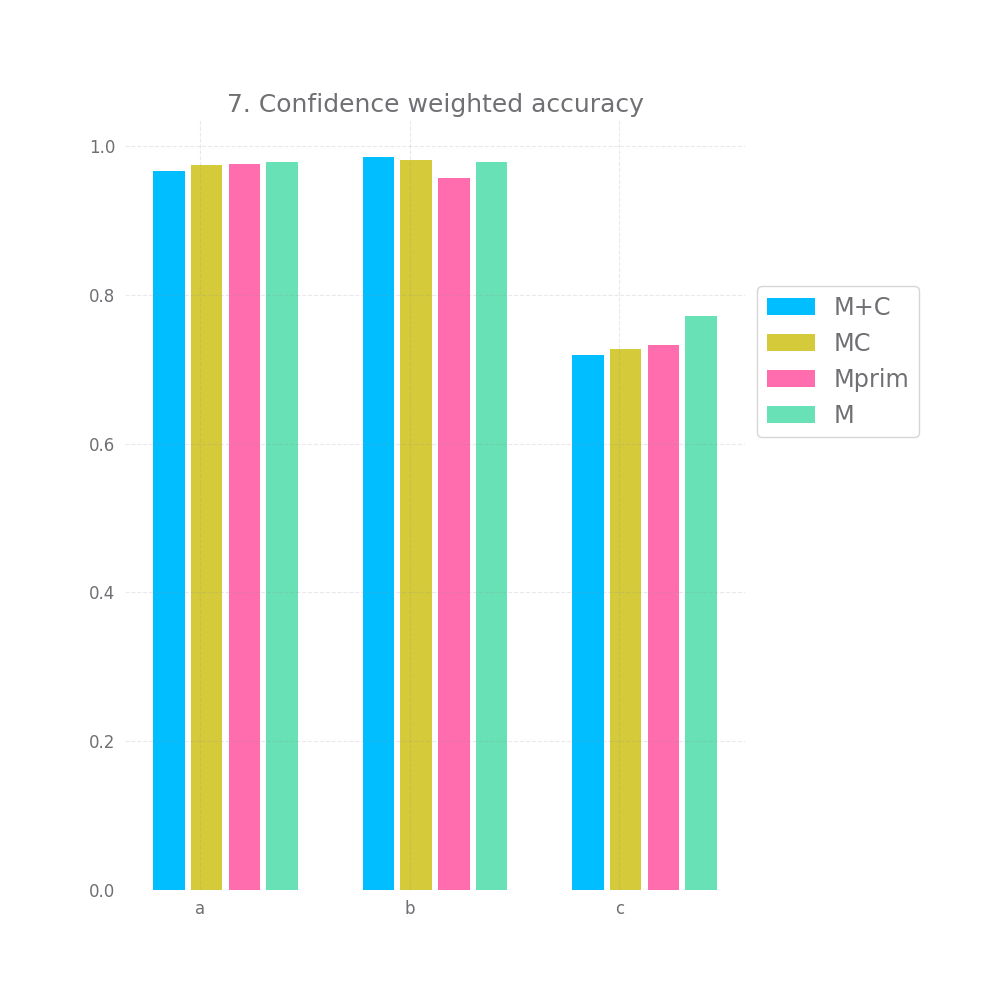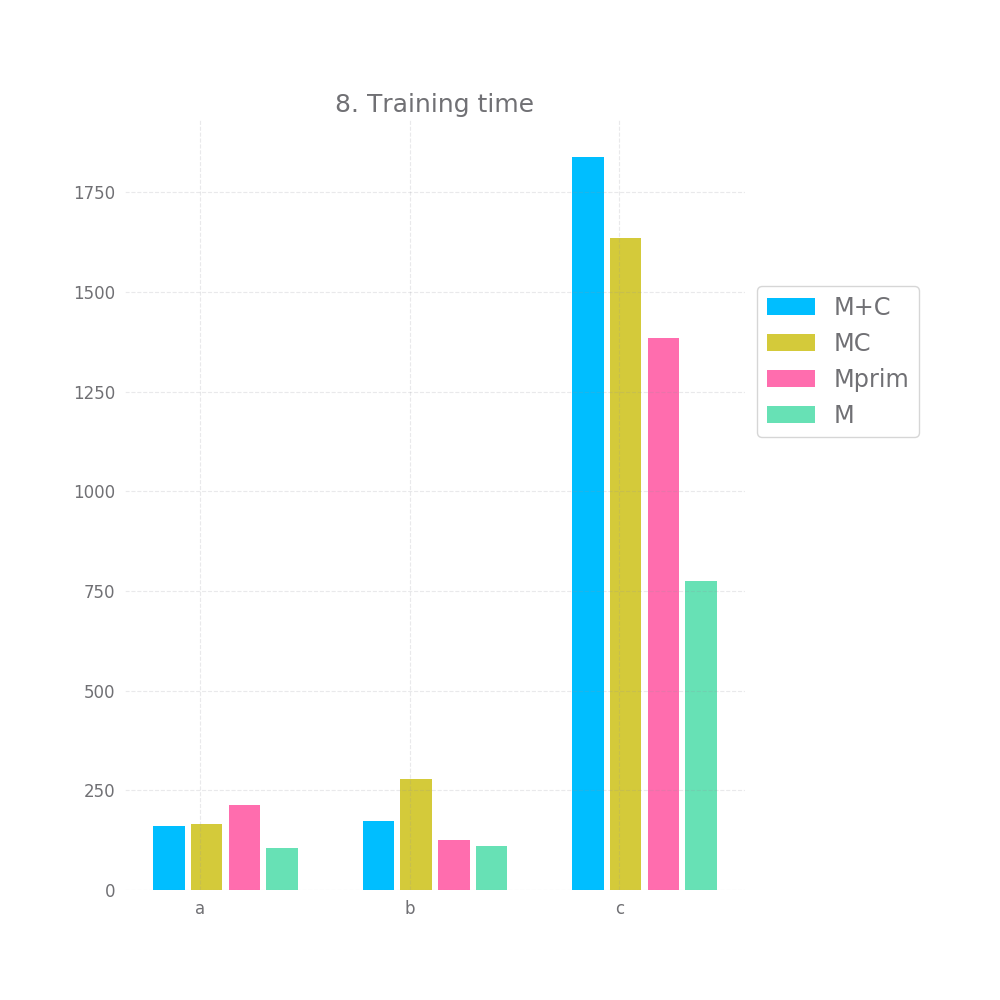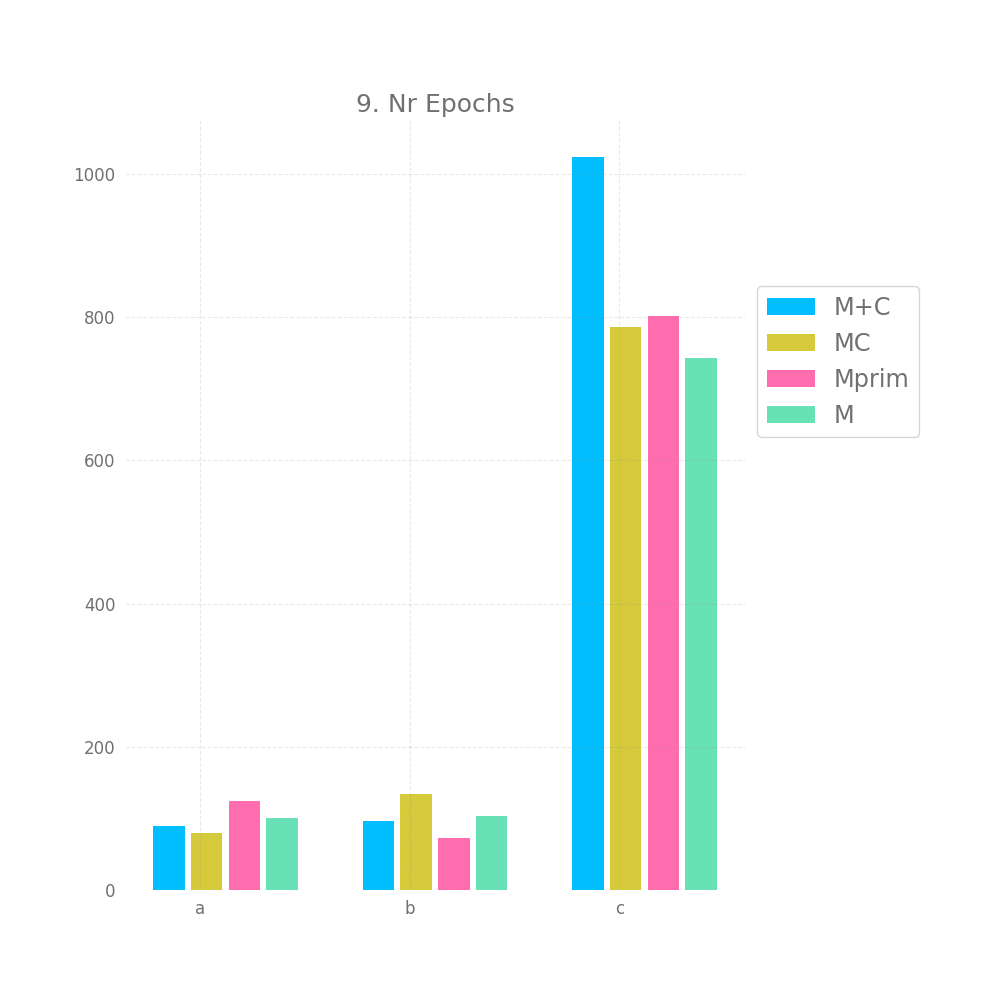Let's explore this one by one:

In terms of raw accuracy, all models performed basically the same, there's some difference, but considering there's some difference between `M+C` and `M`, sometimes with `M`'s accuracy being lower, I think it's safe to think of these small differences as just noise.

This is somewhat disappointing, but to be fair, the models are doing close-to-perfect here anyway, so it could be hard for `Mprim` or `MC` to improve anything. Well, except for the `c` dataset, in theory, one should be able to get an accuracy of ~89% of the `c` dataset, `M`'s accuracy however is only 75%. So one would expect this is where `Mprim` or `MC` could shine, but they don't. There's a `1%` improvement with `Mprim` and even an insignificant drop with `MC` compared to `M`.

There's a bit of hope when we look at graphs nr 2 and 3 measuring the accuracy for high and medium confidence predictions. On all 3 datasets and for all 3 approaches both of these are basically 100%. However, this doesn't hold for graph nr 4, where we take all but the bottom 20% predictions in terms of confidence, here we have a very small accuracy improvement, but it's so tiny it might as well be noise.

Graph 5 and 6 look at how "correct" the confidence is on average. Ideally, the mean confidence should be equal to the accuracy. In that case, both these graphs would be equal to 0.

This is not quite the case here, but it's close enough, we have a difference between 0.2 and 1.4% between the mean confidence and the accuracy... not bad. Also, keep in mind that we test this both on the full testing datasets and a random subset, so this is likely to generalize to any large enough subset of data similar to the training data. Of course, "large enough" and "similar" are basically handwavey terms here.

Lastly, we look at 7 and observe that weighting the accuracy by the confidence doesn't improve accuracy, if anything it seems to make it insignificantly smaller. There might be other ways to observe an effect size here, namely only selecting values with a low confidence, but I'm still rather disappointed by this turnout.

Finally, the training time and number of epochs is unexpected, I'd have assumed Mprim takes the longest to converge, followed by MC and that M+C is the fastest. However, on the `c` dataset we observe the exact opposite. This might serve as some indication that `MC` and `Mprim` can "kind of figure out" the random element earlier, but I don't want to read too much into it.

Let's also take a look at the relative plots: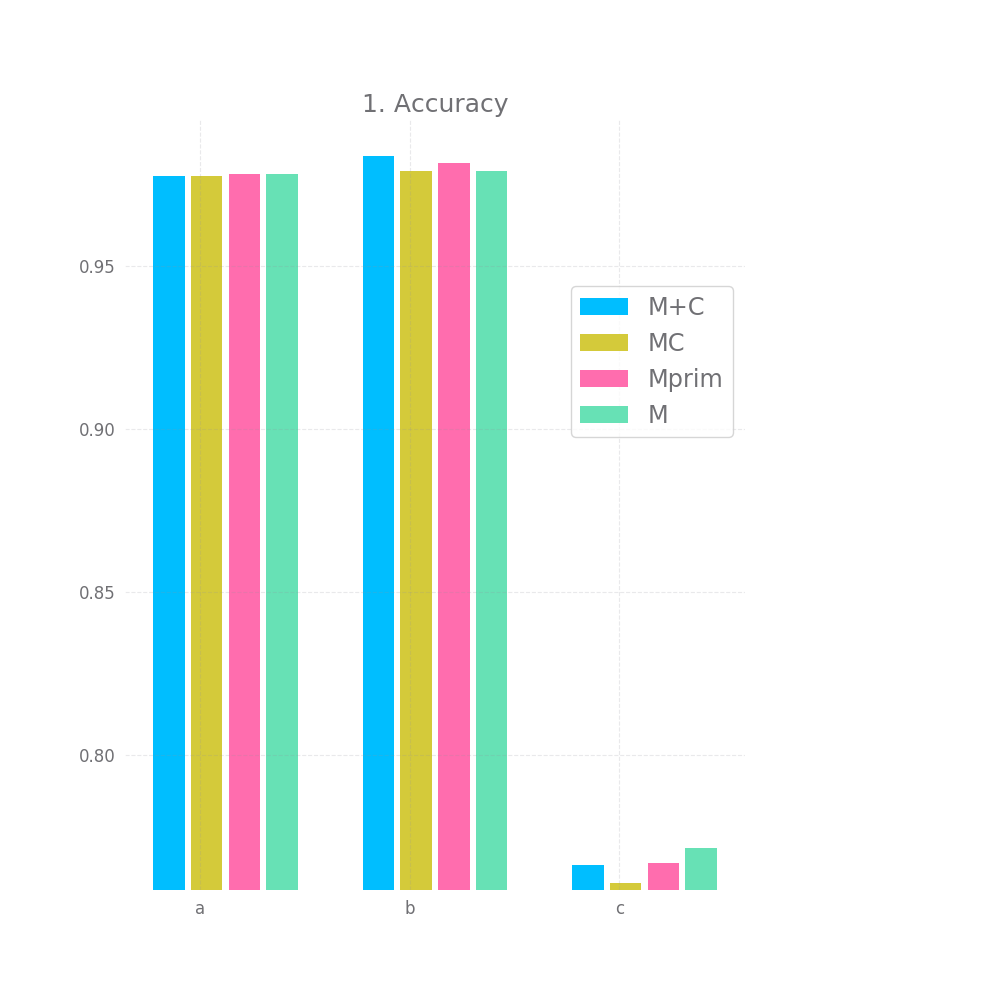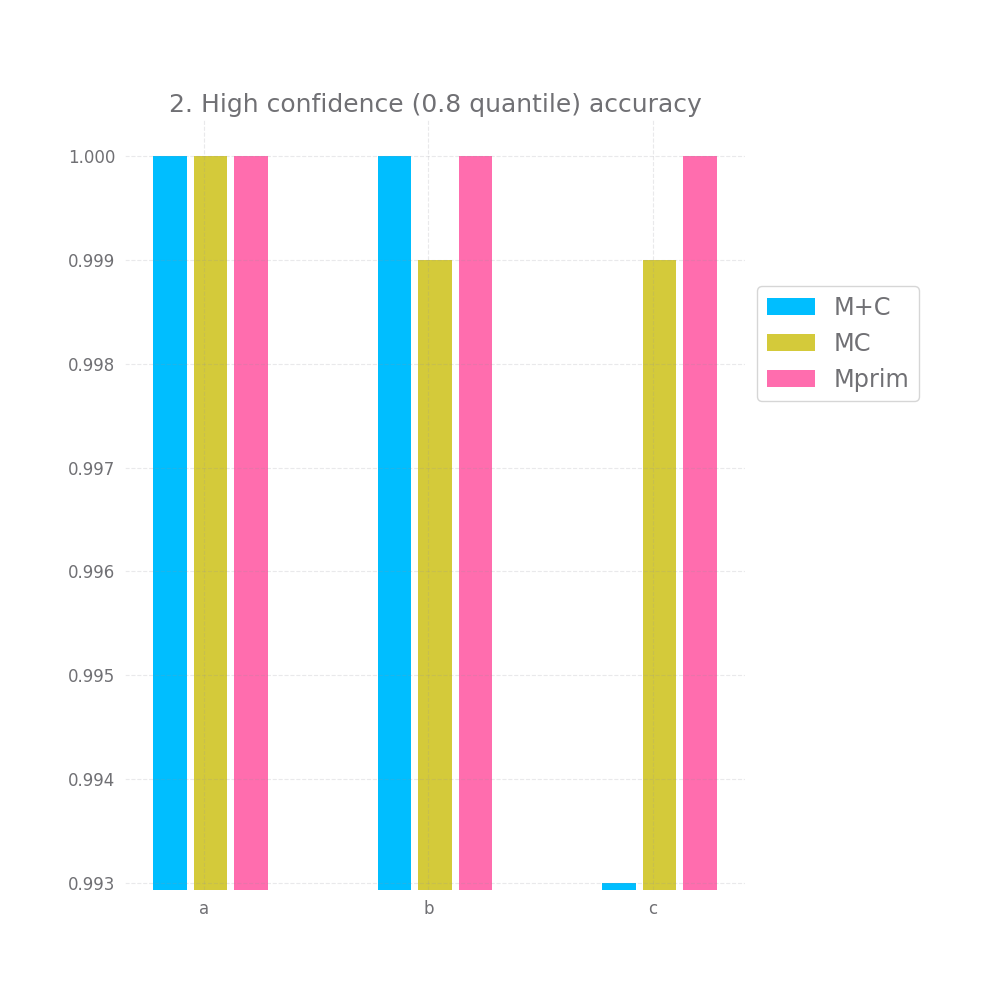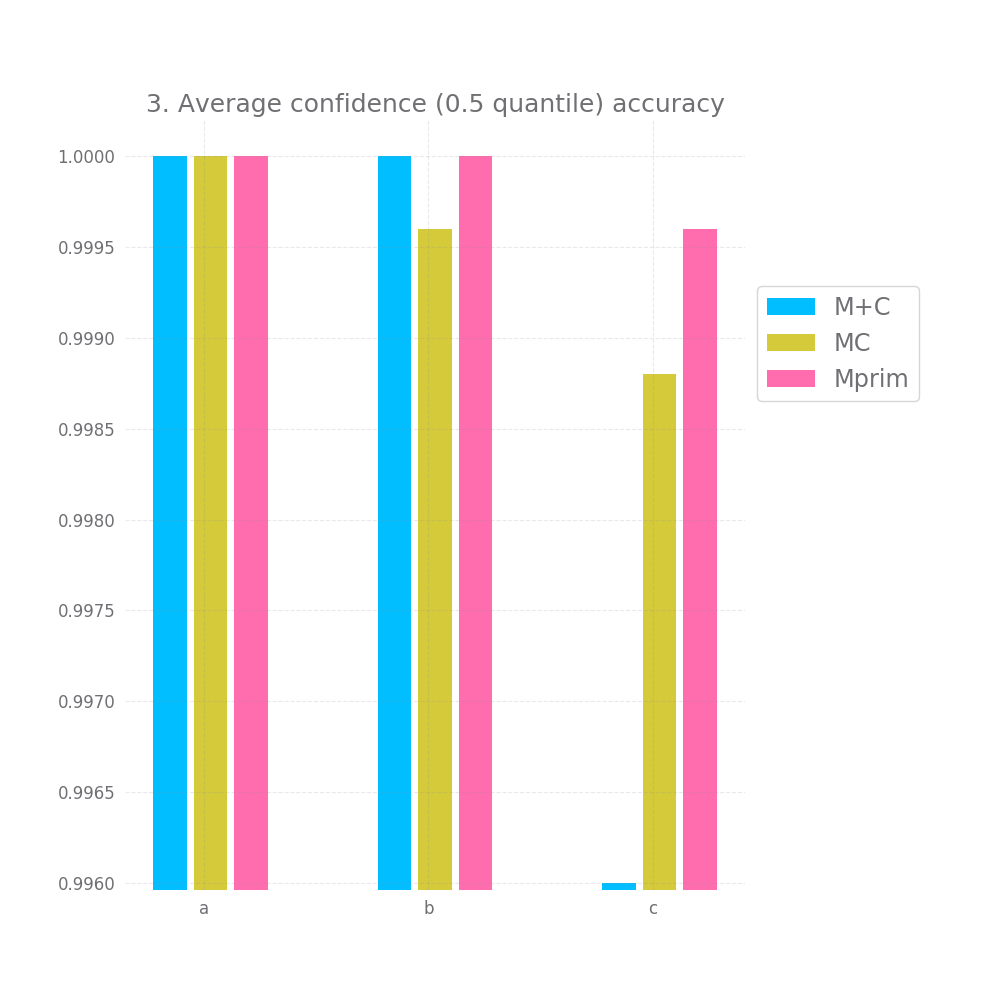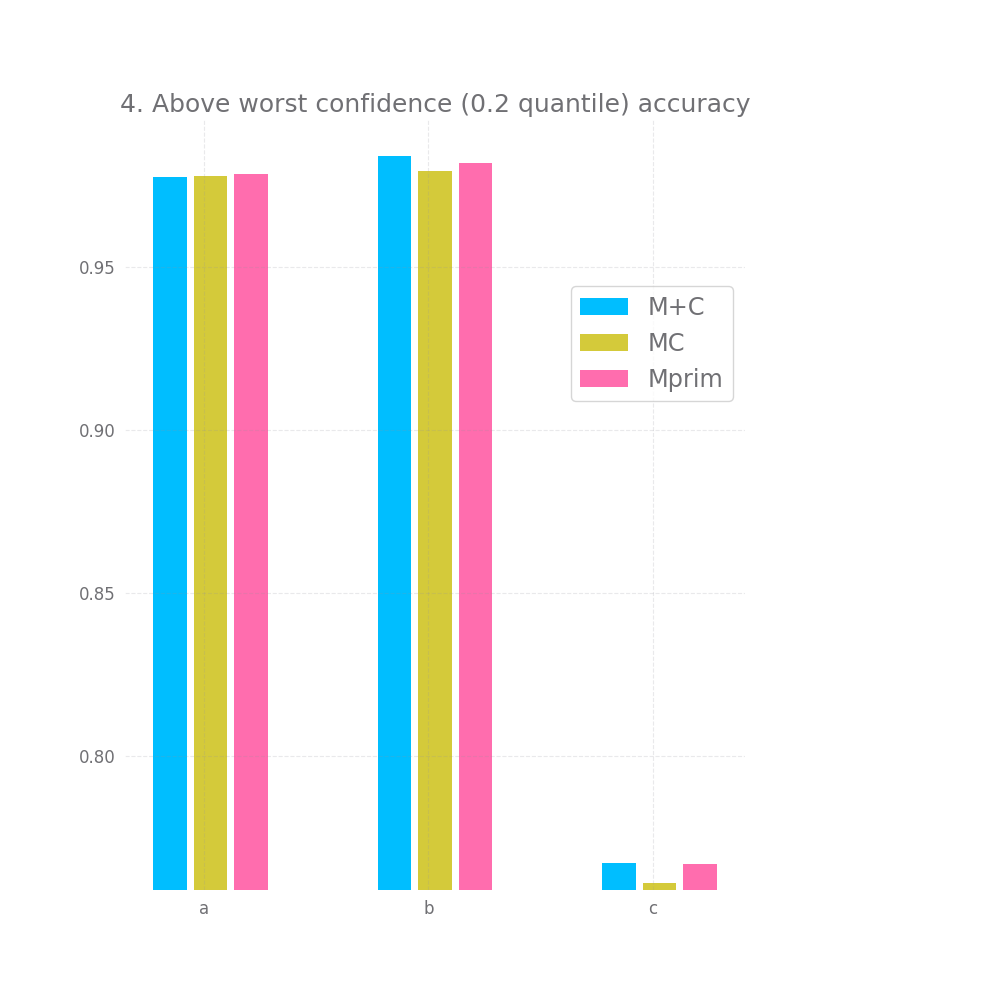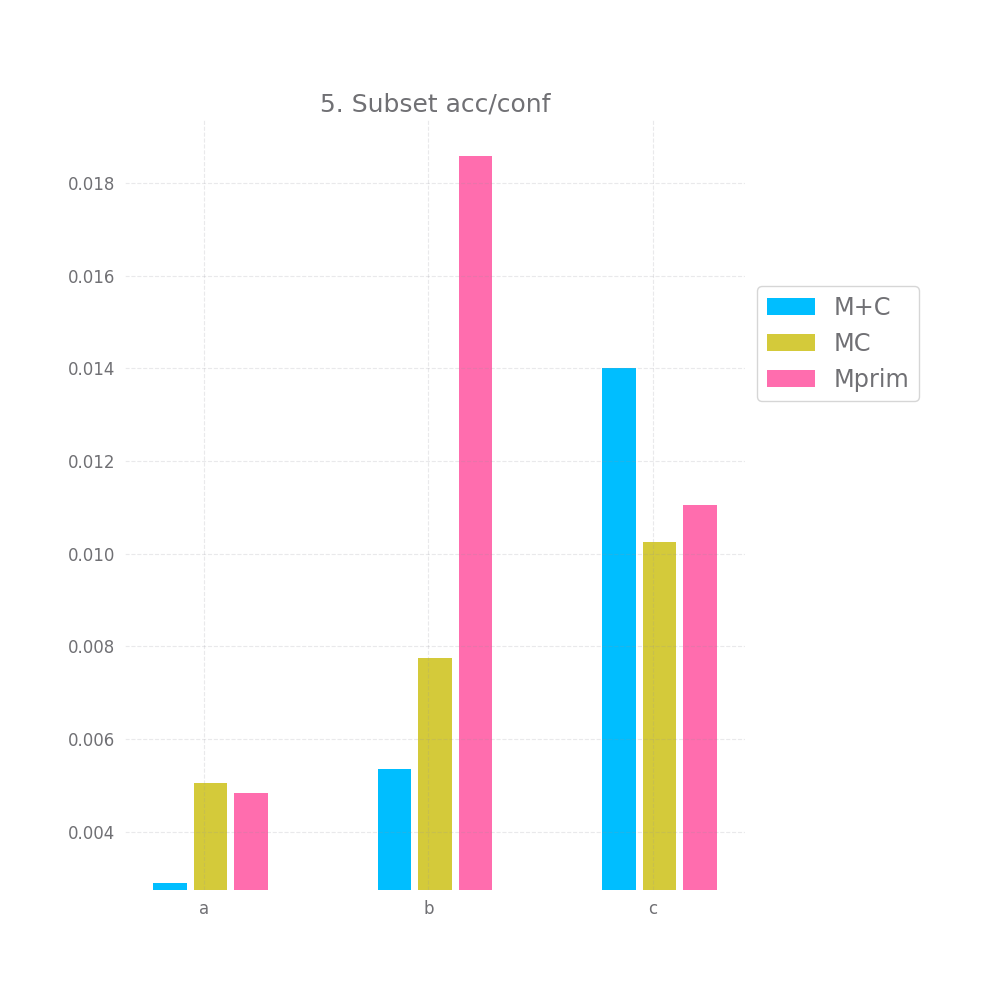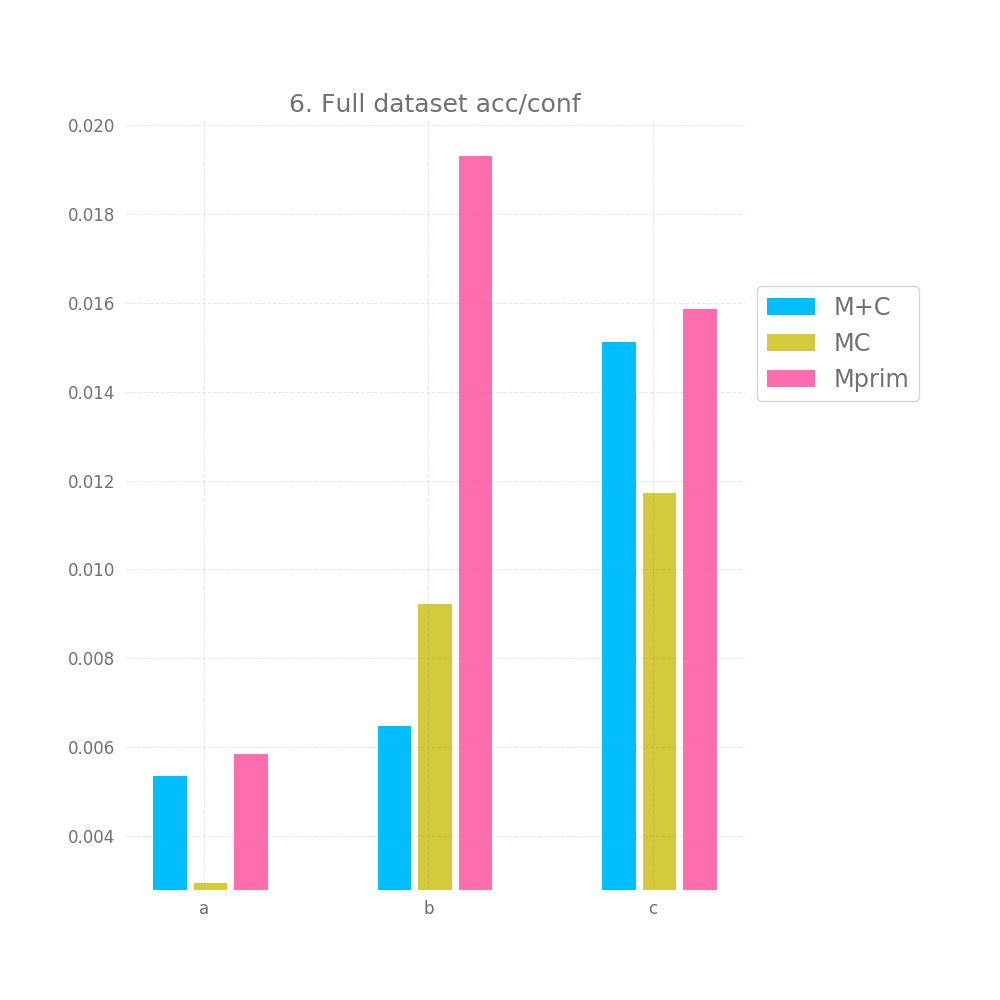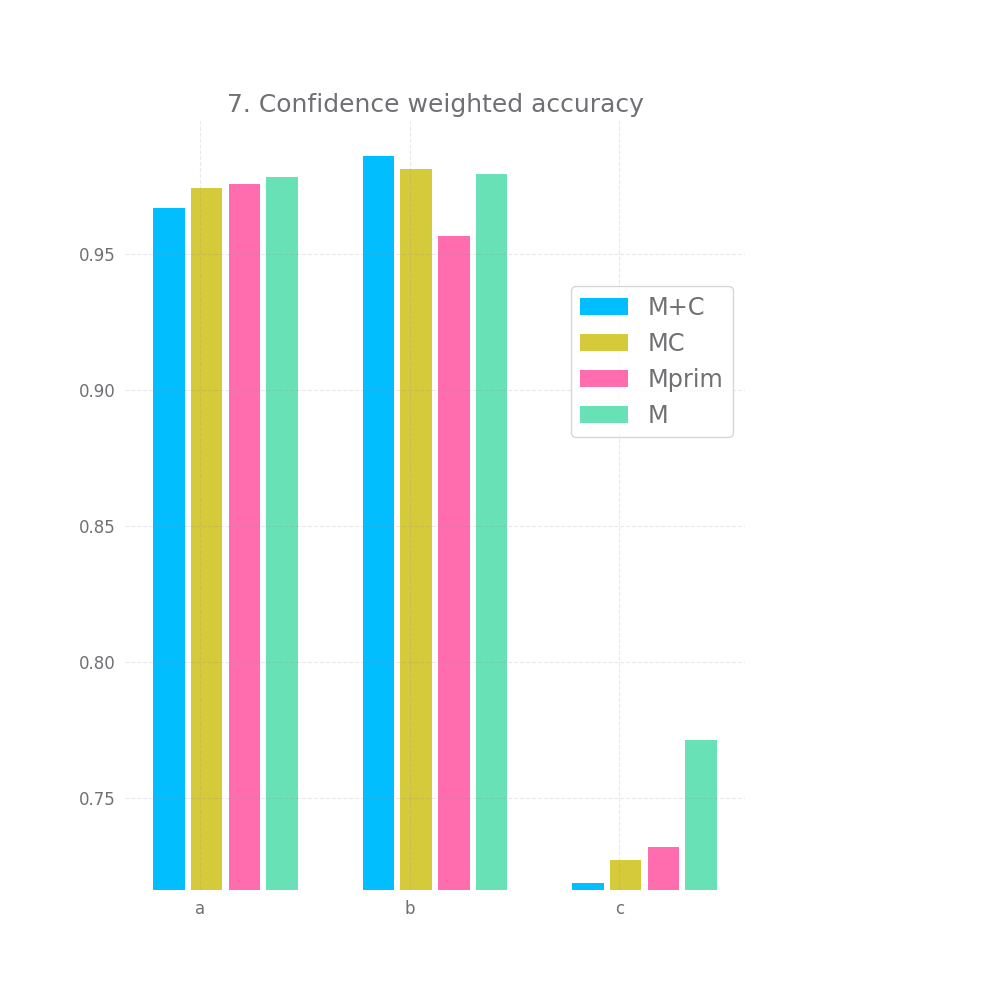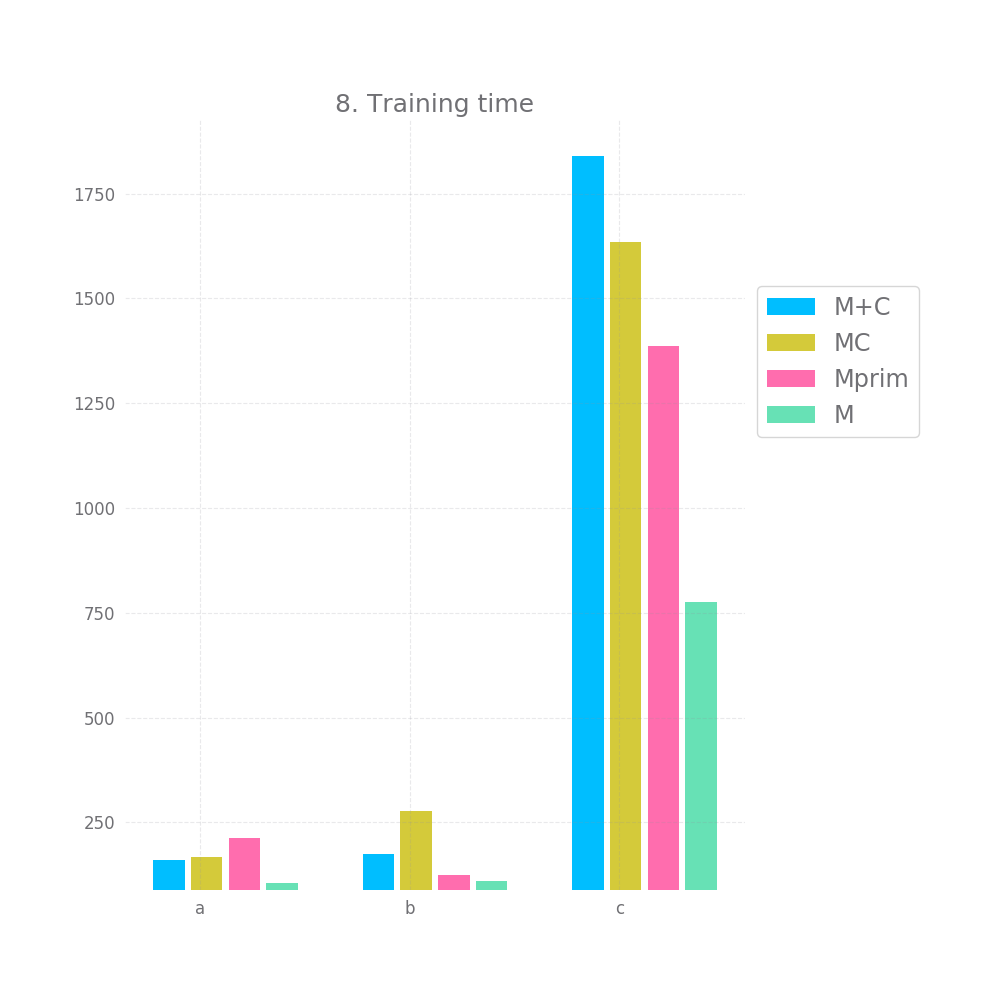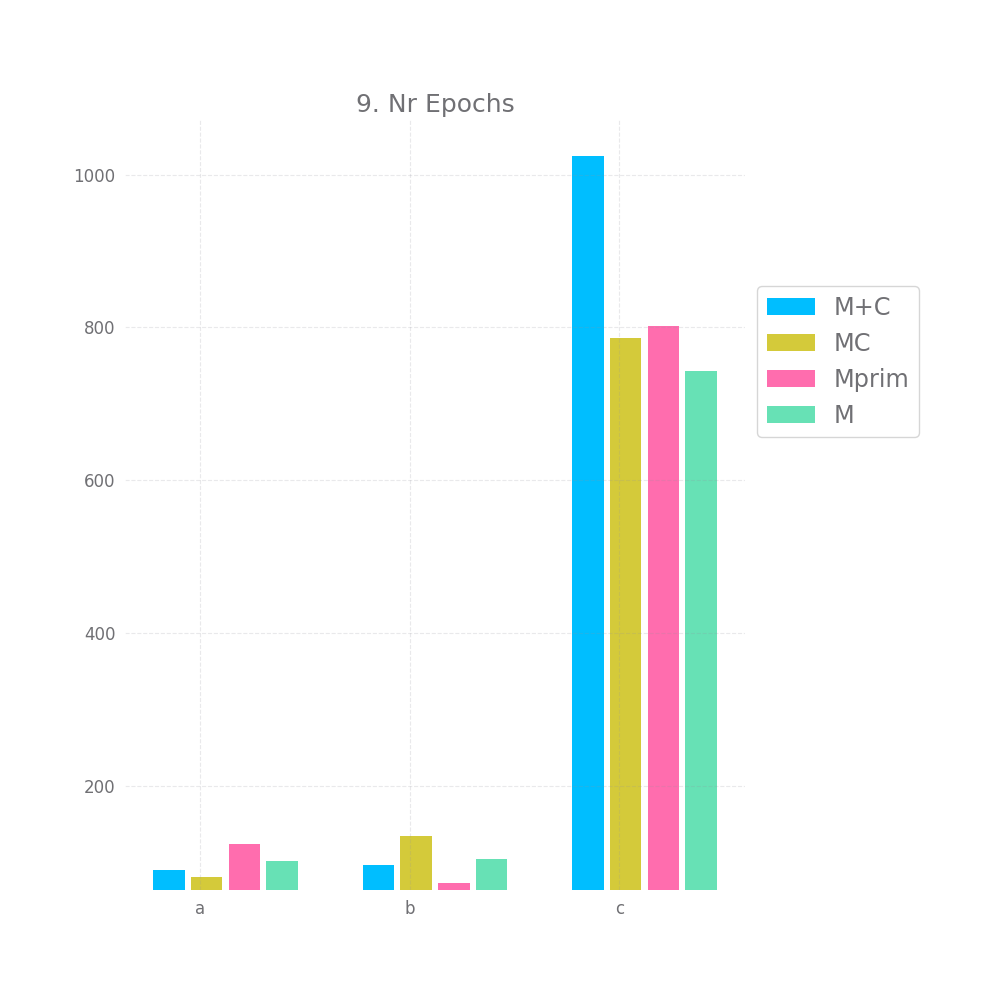## III A broader look at the results

Ok, before I draw any conclusion I also want to do a brief analysis of all the data points I collected. Maybe there's some more signal in all that noise.

Again, remember I have datasets a,b, and c generated using a linear polynomial and polynomial-with-coefficients formula for degrees 3,4,5 and 6. That's 44 more datasets to draw some conclusions from.

I'm going to average out all the scores per model for each dataset and for all datasets combined to see if I find any outliers. I also tried finding surprisingly high scores (e.g. `MC` performing 10% better than `M+C` and `Mprim` on a specific dataset) but couldn't find any, however, feel free to run the code for yourself and dig through my results or generate your own.

That aside being said:

#### 1. On all datasets combined

`M+C` performs better by 1-4% on all accuracy metrics: Accuracy, High confidence accuracy, Average confidence accuracy, Above worst confidence accuracy. Next comes `MC` and `Mprim` is the worst of the bunch by far (`MC` and `M+C` are within 1-2%, `Mprim` is 3-6% away)

`M+C` and `MC` perform about equally on the acc/conf tradeoff both on the whole dataset and on the sample, being off by 2-3.7%. `Mprim` however is much worst and on average it's off by ~23%.

When we "weight" the accuracy using the confidence, the resulting accuracy is still slightly worst for all models compared to the original `M`. However, again, `Mprim` falls behind both `M+C` and `MC`.

#### 2. On dataset of type a

The pattern for the accuracy metric changes a bit, with `MC` being above or about the same as `M+C` and even managing to beat `M` in overall accuracy. But the difference is not that significant

`Mprim` performs horribly, with errors > 45% in the accuracy/confidence tradeoff, both `M+C` and `MC` perform close to perfect, with `M+C` having errors ~3% and `MC` ~0.5%.

The story for weighted accuracy stays the same.

#### 3. On dataset of type b

The pattern for accuracy has `Mprim` and `M+C` tied here, with `MC` being ~4% worst on the overall accuracy and the accuracy of the top 80% of predictions chosen by confidence (i.e. metric 4, above worst confidence).

The acc/conf metric is about the same for all models, nearing a perfect match with errors in the 0.x% and 0.0x%.

The story for weighted accuracy stays the same.

#### 4. On dataset of type c

We've finally reached the good stuff.

This is the only dataset type that is impossible to perfectly predict, this is the kind of situation for which I'd expect confidence models to be relevant.

Average accuracy is abysmal for all models, ranging from 61% to 68%.

When looking at the accuracy of predictions picked by high confidence, for the 0.8 and 0.5 confidence quantile `M+C` has much better accuracy than `Mprim` and `M+C`

For the above worst confidence accuracy (0.2 quantile) all models perform equally badly, at ~73%, but this is still a 5% improvement over the original accuracy.

In terms of the acc/conf metric, `M+C` only has an error of ~2 and ~3%, while `MC` has errors ~11% and `Mprim` has errors of ~22% and ~24%.

Finally, when looking at the confidence weighted accuracy, all 3 confidence models beat the standard accuracy metric, which is encouraging. `MC` does best, but only marginally better than `Mprim`.

However `M`'s accuracy results on datasets of type c were really bad when we compare the confidence weighted accuracy to the plain accuracy of e.g. `MC`, `M+C`, and `Mprim` the confidence weighted accuracy is still bellow the raw accuracy.

## IV Conclusions

To recapitulate, we've tried various datasets that use a multiple-parameter equation to model a 3-way categorial label in order to test various ways of estimating prediction confidence using fully connected networks.

One of the datasets was manipulated to introduce predictable noise in the input->label relationship, the other was manipulated to introduce unpredictable noise in the input->label relationship.

We tried 3 different models:

• `M+C` which is a normal model and a separate confidence predicting network that uses the outputs of `M` and the inputs of `M` as it's own input.

• `MC` which is the same as `M+C` except for the fact that the cost from the `Y` component of the first layer of `C` is propagated through `M`.

• `MPrim` which is a somewhat larger model with an extra output cell representing a confidence value.

It seems that in all 3 scenarios, all 3 models can be useful for selecting predictions with higher than average accuracy and can predict a reliable confidence number, under the assumption that a reliable confidence, when averaged out, should be about equal to the model's accuracy.

On the above task, `MC` and `M+C` performed about equally well, with a potential edge going out to `M+C`. `MPrim` performed significantly worst on many of the datasets.

We did not obtain any significant results in terms of improving the overall accuracy using `MC` and `MPrim`, weighting the accuracy by the confidence did not improve the overall accuracy.

For `MC` and `M+C`, the average confidence matched the average accuracy with a < 0.05 error for the vast majority of datasets, which indicates some "correctness" regarding the confidence.

This is enough to motivate me to do a bit more digging, potentially using more rigorous experiments and more complex datasets on the idea of having a separate confidence-predicting network. Both the `MC` and `M+C` approach seem to be viable candidates here. Outputting confidence from the same model seems to show the worst performance than a separate confidence network.

## V Experimental errors

• Datasets were no varied enough.

• The normal model `M` should have obtained accuracies of ~100% on datasets of type `a` and `b` and ~88% on datasets of type `c`. The accuracies were never quite that high, maybe a completely different result would have been obtained on c), had `M` learned how to predict it as close to reality as possible. However, this behavior would have negated the experimental value for datasets of type `a` and `b`.

• MSELoss was used for the confidence (ups), a linear loss function might have been better here.

• The stopping logic (aka what I've referred to as "model converging") uses and impractically large validation set and it's implementation isn't very straight forward.

• The models were all trained with SDG using a small learning with. We might have obtained better and faster results with a quicker optimizer that used a scheduler.

• Ideally, we would have trained 3 or 5, instead of 1 model per dataset. However, I avoided doing this due to time constraints (the code takes ~10 hour to run on my current machine)

• For metrics like confidence weighted accuracy and especially conf/accuracy on a subset it might have been more correct to CV with multiple subsets. However, since the testing set was separate from the training set, I'm unsure this would have counted as a best practice.

• The terms of the experiments were slightly altered once I realized I wanted to measure more metrics than I had originally planned.

• There are a few anomalies caused by no value being present in the 0.8th quantile of confidence, presumably I should have used a `>=` rather than `>` operator, but now it's too late.

On the whole, if people find this interesting I might run a more thorough experiment taking into account the above points. That being said, it doesn't seem like these "errors" should affect the data relevant for the conclusion too much, though they might be affecting the things presented in section III quite a lot.